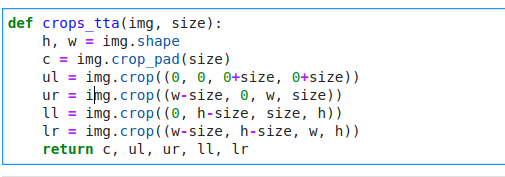# Chapter 7 - Further research Implementing TTA

The further research questions at the end of chapter 7 includes one where you are to use the fastai documentation to build a function that crops an image to a square in each of the four corners, then implement a TTA method that averages the predictions on a center crop and those four crops.

I’ve built the function to get the crops (below) but I’m stuck at implementing the TTA method. How do I get to change the already defined transforms in the datablock to use my TTA function? I thinking of defining the function as a transform but the problem is the output is 5 different crops of the image instead of one for the normal image augmentation transforms.4 Likes

Hey man, I’m pretty much at this exact point right now, have the cropping function but trying to figure out how to implement the TTA method. Is there any direction you can point me in that will help?

Hey @nuggimane,

I ended up implementing the crops as transforms and modifying the batch transforms of the validation dataloader. You can find it here.

Hope it helps.

6 Likes

Thanks I ended up doing something similar at the time but used item transforms instead1 Like

Hey, I wanted to ask if you used this method on Imagenette? I tried my method and the accuracy dropped heavily

1 Like

hey @Arpan, I also remember a drop in accuracy with my implementation. I guess the default approach in fastai is the best to use in practice.

2 Likes

Thanks @kofi for posting your solution. I finally was able to implement tta based on your solution.

But I am curios: how can I make use of the shipped augmentation transforms while using the tta method? You can provide item_tfms and batch_tfms. But I get the error “batch must contain tensors, numpy arrays, numbers, dicts or lists; found <class ‘fastai.vision.core.PILImage’>” when I run the following code.

``learn.tta(n=5, batch_tfms=RandomResizedCrop(size=123))``

The issue here is `RandomResizedCrop` is meant for `PILImages`, not `TensorImages` (which our data are tensor subclasses by the time we get there) as we can see here in RRC’s `encodes`:

So the solution in your case would be to either include it in an `item_tfm` (which that is what it is) or use the GPU equivalent of `RandomResizedCropGPU`

1 Like

Thanks a lot for your hint @muellerzr

I’ve tried both approaches using fastai version 2.1.8
learn.tta(n=5, batch_tfms=RandomResizedCropGPU(size=123))
learn.tta(n=5, item_tfms=RandomResizedCrop(size=123))

But I got TypeError: default_collate: batch must contain tensors, numpy arrays, numbers, dicts or lists; found <class ‘fastai.vision.core.PILImage’> in both cases

From what I can tell in the code, you would need to pass all the item transforms you want, as it overrides it. So IE:

``````item_tfms = [RandomResizedCrop(mysize), ToTensor()]
``````

Or

``````batch_tfms = [RandomResizedCropGPU(mysize), IntToFloatTensor(), Normalize.from_stats(*imagenet_stats)]
``````
1 Like

Thanks a lot @muellerzr. I was able to add RandomResizedCrop based on your help. But now I have some issues using the Zoom transform. Can you give me a hint, on how you would try to find the root cause?

You can see the full code here (and the error in the last cell)

It seems like, that

• the images still have the same size, so Zoom(size=224,…) didn’t do the trick
• Zoom is somehow creating a fifth dimension ( grid with sizes [3, 224, 224, 2])

Zoom is listed under Affine and coord tfm on the GPU, is it not possible to use it in item_tfms then? I’d like to use it there, because I believe I would get most of this TTA approach that way (even a larger RandomResizedCrop in item_tfms would cut away some information).

I checked the source code

• I am not sure if affine_mat is the place where the grid is changed to [3, 224, 224, 2]
• And if it is the root cause, I am not sure how to change it to the shape that I need

Is this snippet equivalent to the TTA implementation in fastai?

``````def ttaify(x: PILImage, k: int) -> TensorImage:
x = ToTensor().encodes(x)

c, h, w = x.shape

top_left     = x[:, : k              ,  :k ]
top_right    = x[:, : k              ,  -k:]
bottom_left  = x[:,  -k:             ,  :k ]
bottom_right = x[:,  -k:             ,  -k:]
centre       = x[:, (h-k)//2:(h+k)//2, (w-k)//2:(w+k)//2]

crops = [top_left, top_right, bottom_left, bottom_right, centre]
avg_img = torch.stack(crops).double().mean(0)

return avg_img``````

This looks pretty good. But as far as I know the “four corners and center TTA” is not implemented in fastai. It was a task for us to see if we can build our own TTA method.

If you want to that, I would suggest that you refactor your code to

• Run `learn.get_preds` on the valid set for each variation of “four corners and center”
• Get the metrics (e.g. accuracy) of each run
• Calcualte an average over all runs
• Run Learner.tta and compare your TTA result to the fastai TTA result

Hello,

Chapter 7 uses `CrossEntropyLossFlat` as the loss function instead of `nn.CrossEntropyLoss`.

When do we use the ‘Flat’ version of the loss function? Is it related to the architecture of the model?

1 Like